Last time I wrote about Python For Loops and If Statements. Today we will talk about how to combine them. In this article, I’ll show you – through a few practical examples – how to combine a for loop with another for loop and/or with an if statement!

Note: This is a hands-on tutorial. I highly recommend doing the coding part with me – and if you have time, solving the exercises at the end of the article! If you haven’t done so yet, please work through these articles first:

Note 2: On mobile the line breaks of the code snippets  might look tricky. But if you copy-paste them into your Jupyter Notebook, you will see the actual line breaks much clearer!

## For loop within a for loop – aka the nested for loop

The more complicated the data project you are working on, the higher the chance that you will bump into a situation where you have to use a nested for loop. This means that you will run an iteration, then another iteration inside that iteration.

Let’s say you have nine TV show titles put into three categories: comedies, cartoons, dramas. These are presented in a nested Python list (“lists in a list”):

```my_movies = [['How I Met Your Mother', 'Friends', 'Silicon Valley'],
['Family Guy', 'South Park', 'Rick and Morty'],
['Breaking Bad', 'Game of Thrones', 'The Wire']]
```

You want to count the characters in all these titles and print the results one by one to your screen, in this format:

`"The title [movie_title] is [X] characters long."`

How would you do that? Since you have three lists in your main list, to get the movie titles, you have to iterate through your `my_movies` list — and inside that list, through every sublist, too:

```for sublist in my_movies:
for movie_name in sublist:
char_num = len(movie_name)
print("The title " + movie_name + " is " + str(char_num) + " characters long.")
```

Note: remember `len()` is a Python function that results in an integer. To put this integer into a “printable” sentence, we have to turn it into a string first. I wrote about this in the previous Python For Loops tutorial.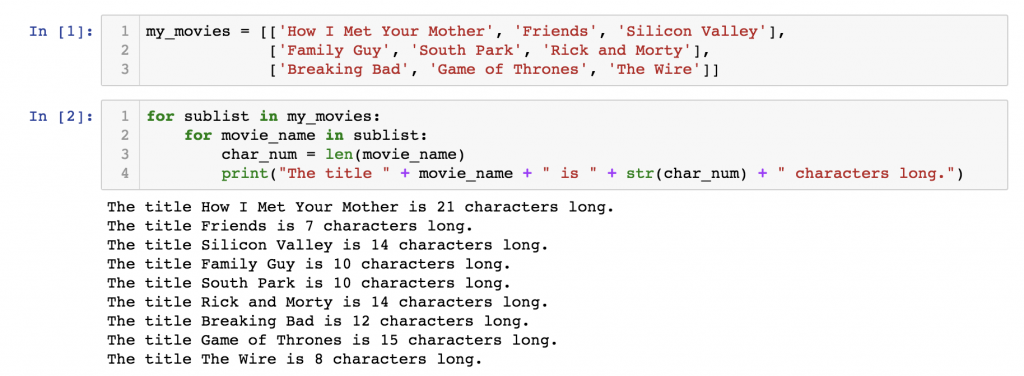I know, Python for loops can be difficult to understand for the first time… Nested for loops are even more difficult. If you have trouble understanding what exactly is happening above, get a pen and a paper and try to simulate the whole script as if you were the computer — go through your loop step by step and write down the results.

One more thing:
Syntax! The rules are the same ones you learned when we discussed simple for loops — the only thing that I’d like to emphasize, and that you should definitely watch out for, is the indentations. Using proper indentations is the only way how you can let Python know that in which for loop (the inner or the outer) you would like to apply your block of code. Just test out and try to find the differences between these three examples:Example 1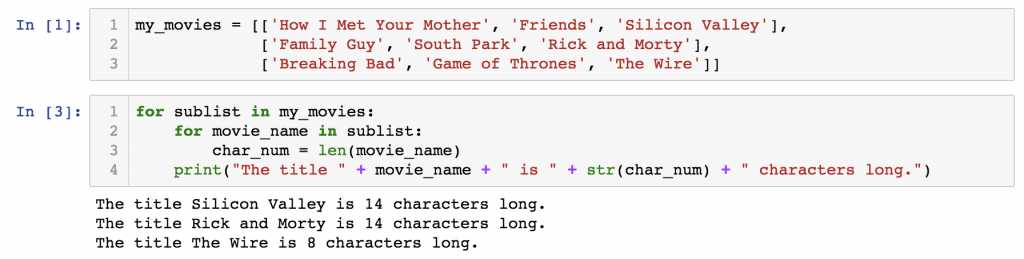Example 2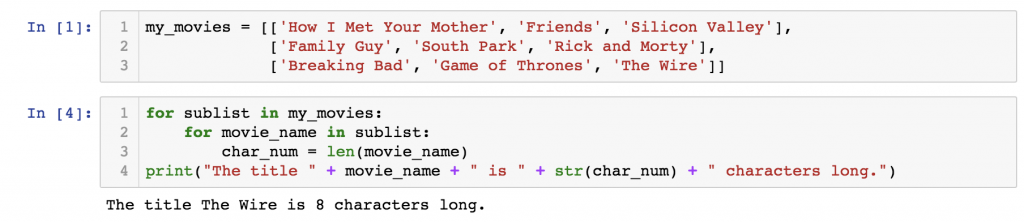Example 3

## If statement within a for loop

Inside a for loop, you can use if statements as well.

Let me use one of the most well-known examples of the exercises that you might be given as the opening question in a junior data scientist job interview.

Go through all the numbers up until 99. Print ‘fizz’ for every number that’s divisible by 3, print ‘buzz’ for every number divisible by 5, and print ‘fizzbuzz’ for every number divisible by 3 and by 5! If the number is not divisible either by 3 or 5, print a dash (‘-‘)!

Here’s the solution!

```for i in range(100):
if i % 3 == 0 and i % 5 == 0:
print('fizzbuzz')
elif i % 3 == 0:
print('fizz')
elif i % 5 == 0:
print('buzz')
else:
print('-')
```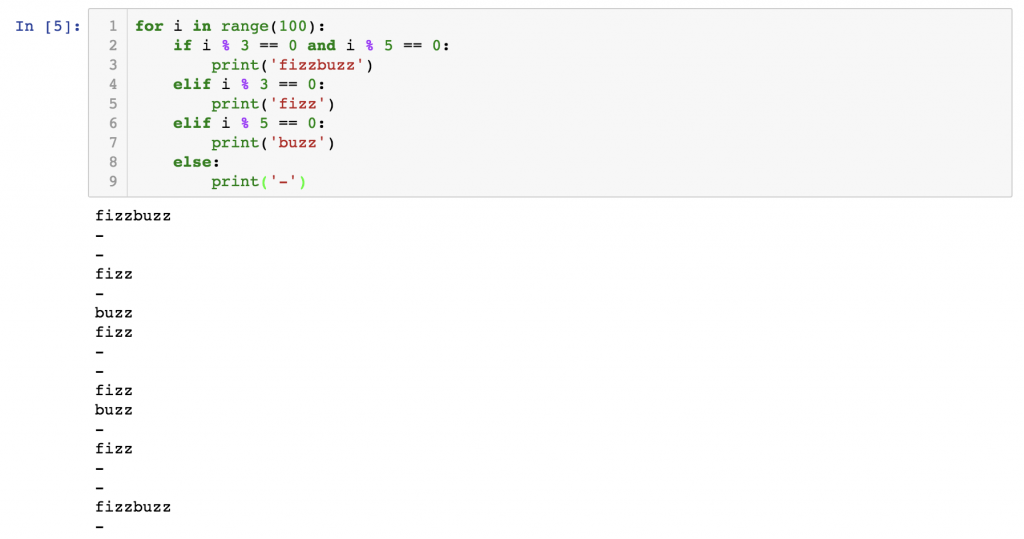As you can see, an if statement within a for loop is perfect to evaluate a list of numbers in a range (or elements in a list) and put them into different buckets, tag them, or apply functions on them – or just simply print them.

Again: when you use an if statement within a for loop, be extremely careful with the indentations because if you misplace them, you can get errors or fake results!

## Break

There is a special control flow tool in Python that comes in handy pretty often when using if statements within for loops. And this is the `break` statement.

Can you find the first 7-digit number that’s divisible by 137? (The first one and only the first one.)

Here’s one solution:

```for i in range(0, 10000000, 137):
if len(str(i)) == 7:
print(i)
break
```

This loop takes every 137th number (`for i in range(0, 10000000, 137)`) and it checks during each iteration whether the number has 7 digits or not (`if len(str(i)`) == 7). Once it gets to the the first 7-digit number, the if statement will be `True` and two things happen:

1. `print(i)` –» The number is printed to the screen.
2. `break` breaks out of the for loop, so we can make sure that the first 7-digit number was also the last 7-digit number that was printed on the screen.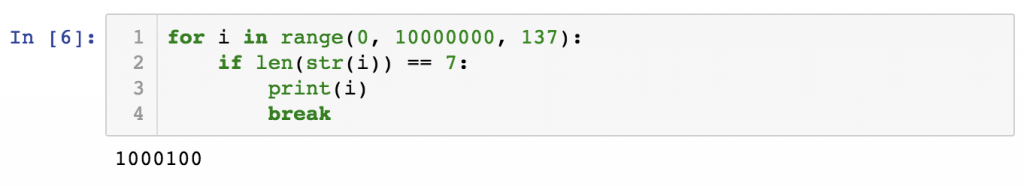Learn more about the `break` statement (and its twin brother: the `continue` statement) in the original Python3 documentation: here.

Note: you can solve this task more elegantly with a while loop. However, I haven’t written a while loop tutorial yet, which is why I went with the for loop + `break` solution!

## Test Yourself!

It’s time to test whether you have managed to master the if statement, the for loops and the combination of these two! Let’s try to solve this small test assignment!

Create a Python script that finds out your age in a maximum of 8 tries! The script can ask you only one type of question: guessing your age! (e.g. “Are you 67 years old?”) And you can answer only one of these three options:

• less
• more
• correct

Based on your answer the computer can come up with another guess until it finds out your exact age.

Note: to solve this task, you will have to learn a new function, too. That’s the `input()` function! More info: here.

## Solution

Here’s my code.

Note 1: One can solve the task with a while loop, too. Again: since I haven’t written about while loops yet, I’ll show you the for loop solution.
Note 2: If you have an alternative solution, please do not hesitate to share it with me and the other readers in the comment section below!

```down = 0
up = 100
for i in range(1,10):
guessed_age = int((up + down) / 2)
answer = input('Are you ' + str(guessed_age) + " years old?")
print("Nice")
break
up = guessed_age
down = guessed_age
else:
```

My logic goes:
STEP 1) I set a range between 0 and 100 and I assume that the age of the “player” will be between these two values.
`down = 0`
`up = 100`

STEP 2) The script always asks the middle value of this range (for the first try it’s `50`):

```guessed_age = int((up + down) / 2)
answer = input('Are you ' + str(guessed_age) + " years old?")
```

STEP 3) Once we have the “player’s” answer, there are four possible scenarios:

• If the guessed age is correct, then the script ends and it returns some answer.
```if answer == 'correct':
print("Nice")
break
```
• If the answer is “less”, then we start the iteration over – but before that we set the maximum value of the age-range to the guessed age. (So in the second iteration the script will guess the middle value of 0 and 50.)
```elif answer == 'less':
up = guessed_age
```
• We do the same for the “more” answer – except that in this case we change the minimum (and not the the maximum) value:
```elif answer == 'more':
down = guessed_age
```
• And eventually we handle the wrong answers and the typos:
```else: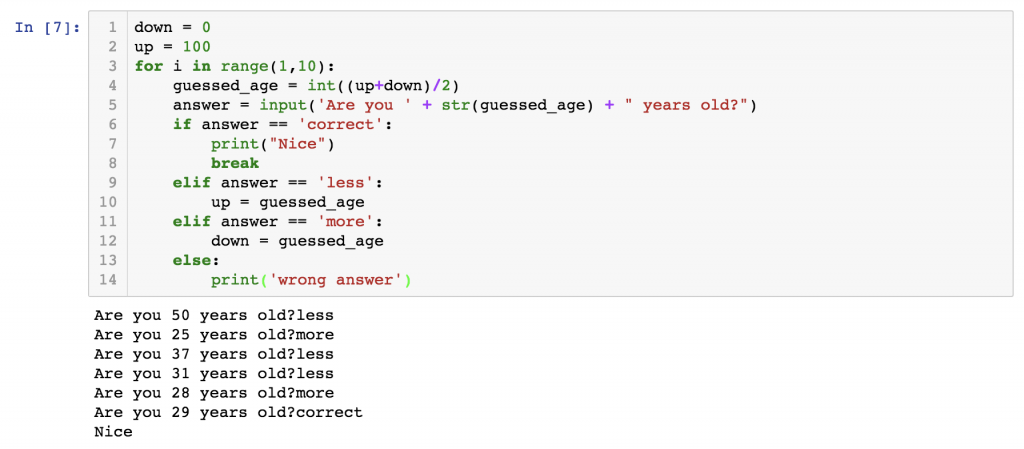Did you find a better solution?
Share it with me in the comment section below!

## Conclusion

Now you’ve got the idea of:

• Python nested for loops and
• for loops and if statements combined.

They are not necessarily considered to be Python basics; this is more like a transition to the intermediate level. Using them requires a solid understanding of Python3’s logic – and a lot of practicing, too.

There are only two episodes left from the Python for Data Science Basics tutorial series! Keep it going and continue with the Python syntax essentials!

Cheers,
Tomi